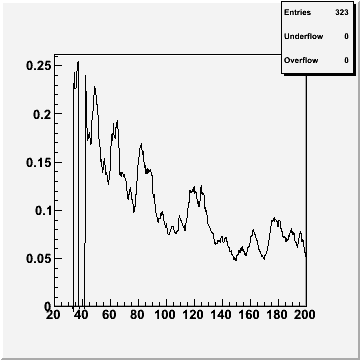# Histogram exclude zero

Hello Rooters,

I am trying to draw the contents of a TNtupleD with a condition. When the condition is false (the point beeing 0 then), then the line should go through the next point not going through the point at 0.

As I understand the Drawing Option:
“P” : Draw current marker at each bin except empty bins
will do that for the markers.
Is there a similar way to also exlude these points also for the drawn line?

The code I am using is the following:

``` WithNoise->Draw("Height>>h11(360,20,200)","ErrorCoM*(ErrorCoM < 0.25)","c"); TH1D* h11 = (TH1D*)gDirectory->Get("h11"); h11->SetTitle(""); ```
As you can see in the attached picture, the line goes down to zero when the condition is false. How can I avoid that and instead make the line go through the next point where the condition is true again?

Thank you,
LutzHere is a possible way to get a continuous graph from an histogram having bins with zero contanet.

``````{
Int_t nb = hpx->GetNbinsX();
Double_t x,y;
TGraph *g = new TGraph();
Int_t ig=0;
for (Int_t i=1; i<=100; i++) {
y = hpx->GetBinContent(i);
x = hpx->GetXaxis()->GetBinCenter(i);
if (y>0) {
g->SetPoint(ig,x,y);
ig++;
}
}
TCanvas c;
c->Divide(1,2);
c->cd(1); hpx->Draw();
c->cd(2); g->Draw("al*");
}``````